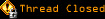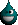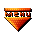# Topic awaiting preservation: Calculation problem (Page 1 of 1)Hiroki
Paranoid (IV) Inmate

From: NZ
Insane since: Dec 2002posted 11-06-2003 04:38

Hi, guys. How are you?
I am strugling calculating values of text box.
I generated input text boxes inside of the loop.
They are named price and quant.
Then I wanted to do like price * quant = cost when I click calcualte button, you know.
and print on the page.
But some reason, it just say: NaN, NaN, Nan......
Hmm.....Here is my code:

code:
```<html>
<title>Shop Form</title>
<script type="text/javascript">
function calculate(){
var price = parseInt(document.theForm.price.value);
var quant = parseInt(document.theForm.quant.value);
var total = 0;
for(i=1; i<=10; i++){
total=price*quant;
document.write(total);
}
}
</script>
<body>
<form name="theForm">
<script type="text/javascript">
for(i=1; i<=10; i++){
document.write("<input type='text' name='price' value='0'>" );
document.write("<input type='text' name='quant' value='0'>" );

document.write("<br >");
}
</script>
<input type="button" onClick="calculate()" value="Get total">
</form>
</body>
</html>```

I haven't done JavaScript for a while.....
Easy to forget...........
Help.

Hiroki Kozai

Bipolar (III) Inmate

From: Israel
Insane since: Nov 2001posted 11-06-2003 19:42

1) You refer to document.theForm.price.value / document.theForm.quant.value where you actually have 10 instances per each name therefore you should refer to the elements as following:
var prices = document.getElementsByName("price");
var quants = document.getElementsByName("quant");
and during the iteration you can get the values of the price and quant by using the
var price = parseInt(prices.item(i-1).value);
var quant = parseInt(quants.item(i-1).value);

2) You are trying to write the total using the document.write(total); which will illuminate the whole document content and you will get runtime exception for referring nonsexist elements. You can define another text box for the total (document.write("<input type='text' name='total' value='' disabled>" )and put the result into.

Hiroki
Paranoid (IV) Inmate

From: NZ
Insane since: Dec 2002posted 11-06-2003 21:05

Hi, Yossi. Thank you very much.
It worked!
Have a good day , mate.
Cya.

Hiroki Kozai